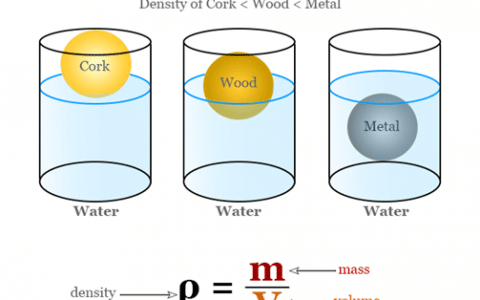# Tag: what is the formula for calculating density## Calculating Density of any matter around us

The density of matter is simply defined as mass per unit volume. Density means the amount of matter present in…